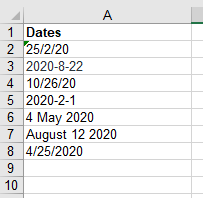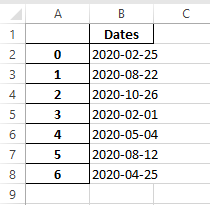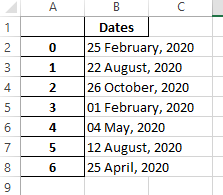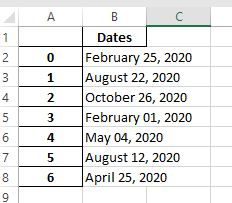# Python Program to Convert any Dates in Spreadsheets

In this tutorial, we’ll look at how to use Python to convert any Dates in Spreadsheets.

Let us take an example of demo.xlsx excel spreadsheet that is shown below:This file contains a single column called ‘Dates,’ which stores random dates from 2020 in various formats.

to_datetime() Method: To conduct date and time operations, we must first convert the column to a datetime data type.

dt.strftime() Method:

Then, using a value of “%Y-%m-%d” we utilise the dt and strftime methods to tell Python how to format the date. The example we used here is “% Y-% m-% “, where % Y is the whole year, % m is the month with two digits, and % d is the day with two digits.

## Program to Convert any Dates in Spreadsheets in Python

Example1

Approach:

• Import pandas module as pd using the import keyword.
• Read the file and indicate which column contains the Dates using the read_excel() function and store it in a variable.
• (here the file has only 1 column, hence we didn’t specify Dates column).
• Convert the Dates column in the above file to YYYY-MM-DD format using the to_datetime,dt.strftime functions.
• And store the formated dates output into another Excel file using the to_excel() function.
• The Exit of the Program.

Below is the implementation:

```# Import pandas module as pd using the import keyword
import pandas as pd

# Read the file and indicate which column contains the Dates using the read_excel() function
# and store it in a variable
# (here the file has only 1 column, hence we didn't specify Dates column)

# Convert the Dates column in the above file to YYYY-MM-DD format using the to_datetime,dt.strftime functions
# And store the formated dates output into another Excel file using the to_excel() function.
demo_file["Dates"] = pd.to_datetime(
demo_file["Dates"]).dt.strftime("%Y-%m-%d")
demo_file.to_excel("demo_format.xlsx")```

Output:Different date formats:
Example: Monday, 16 May, 2020, 5:00 PM

“%A, %B %d” -> “Monday, May18”
“%d-%b-%y” -> “16-May-20”
“%d/%m/%Y” -> “16/05/2020”
“%b %d, %Y” -> “May 16, 2020”

 Directive Description Example %a Weekday as locale’s abbreviated name Sun, Mon,….,Sat(en_US); So, Mo,……, Sa(de_DE) %A Weekday as locale full name Sunday, Monday, Tuesday,….., Saturday %w Weekday as a decimal number, where 0 represents Sunday and 6 represents Saturday 0, 1, 2, 3, 4, 5, 6 %d The month’s day as a zero-padded decimal number 01, 02, 03, …………,31

Example2:

Now let us change the date format as shown below for the same dataset

Format:

` "%d %b, %Y"  --> "16 May, 2020"`
```# Import pandas module as pd using the import keyword
import pandas as pd

# Read the file and indicate which column contains the Dates using the read_excel() function
# and store it in a variable
# (here the file has only 1 column, hence we didn't specify Dates column)

# Convert the Dates column in the above file to "D MMMM, YYYY" format using the to_datetime,dt.strftime functions
# And store the formated dates output into another Excel file using the to_excel() function.
demo_file["Dates"] = pd.to_datetime(
demo_file["Dates"]).dt.strftime("%#d %B, %Y")
demo_file.to_excel("demo_format.xlsx")
```

Output:Example3

Now let us change the date format as shown below for the same dataset:

Format:

`"%B %d, %Y" --> "May 16, 2020"`
```# Import pandas module as pd using the import keyword
import pandas as pd

# Read the file and indicate which column contains the Dates using the read_excel() function
# and store it in a variable
# (here the file has only 1 column, hence we didn't specify Dates column)